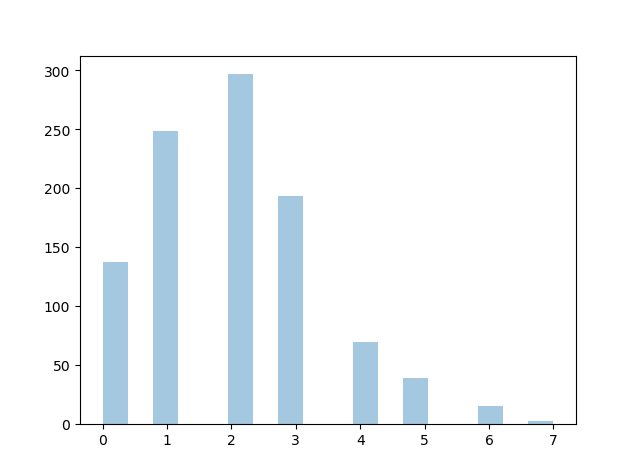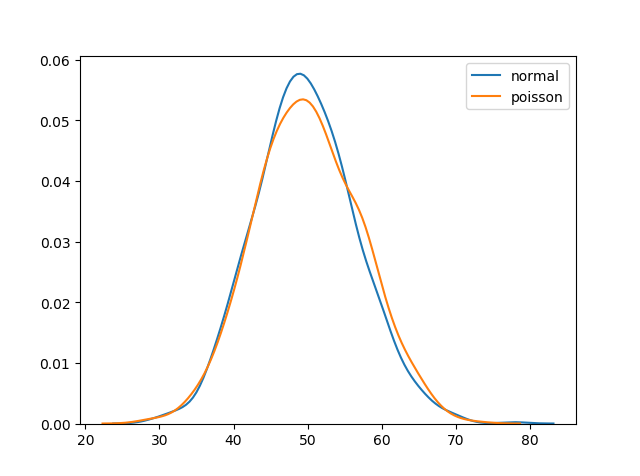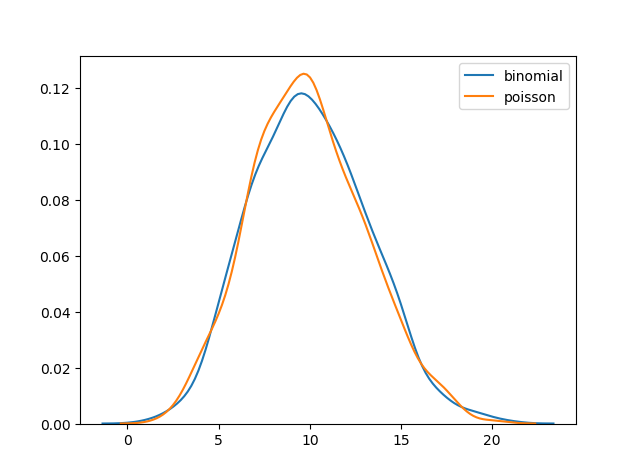THE WORLD'S LARGEST WEB DEVELOPER SITE

# Poisson Distribution

## Poisson Distribution

Poisson Distribution is a Discrete Distribution.

It estimates how many times an event can happen in a specified time. e.g. If someone eats twice a day what is probability he will eat thrice?

It has two parameters:

`lam` - rate or known number of occurences e.g. 2 for above problem.

`size` - The shape of the returned array.

### Example

Generate a random 1x10 distribution for occurence 2:

from numpy import random

x = random.poisson(lam=2, size=10)

print(x)
Try it Yourself »

## Visualization of Poisson Distribution

### Example

from numpy import random
import matplotlib.pyplot as plt
import seaborn as sns

sns.distplot(random.poisson(lam=2, size=1000), kde=False)

plt.show()

### ResultTry it Yourself »

## Difference Between Normal and Poisson Distribution

Normal distribution is continous whereas poisson is discrete.

But we can see that similar to binomial for a large enough poisson distribution it will become similar to normal distribution with certain std dev and mean.

### Example

from numpy import random
import matplotlib.pyplot as plt
import seaborn as sns

sns.distplot(random.normal(loc=50, scale=7, size=1000), hist=False, label='normal')
sns.distplot(random.poisson(lam=50, size=1000), hist=False, label='poisson')

plt.show()

### ResultTry it Yourself »

## Difference Between Poisson and Binomial Distribution

The difference is very subtle it is that, binomial distribution is for discrete trials, whereas poisson distribution is for continuous trials.

But for very large `n` and near-zero `p` binomial distribution is near identical to poisson distribution such that `n * p` is nearly equal to `lam`.

### Example

from numpy import random
import matplotlib.pyplot as plt
import seaborn as sns

sns.distplot(random.binomial(n=1000, p=0.01, size=1000), hist=False, label='binomial')
sns.distplot(random.poisson(lam=10, size=1000), hist=False, label='poisson')

plt.show()

### ResultTry it Yourself »